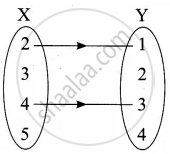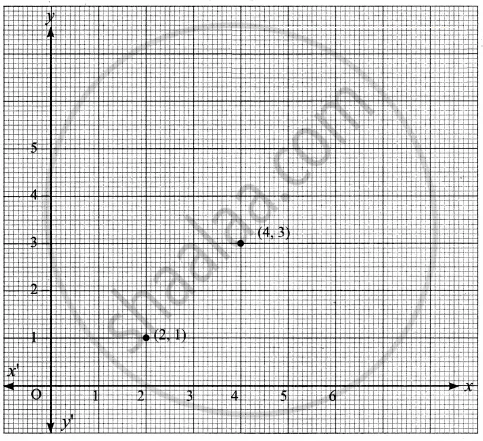Tamil Nadu Board of Secondary EducationSSLC (English Medium) Class 10th

# Represent the given relation by (a) an arrow diagram(b) a graph and (c) a set in roster form, wherever possible {(x, y) | x = 2y, x ∈ {2, 3, 4, 5}, y ∈ {1, 2, 3, 4} - Mathematics

Diagram
Graph
Sum

Represent the given relation by
(a) an arrow diagram
(b) a graph and
(c) a set in roster form, wherever possible

{(x, y) | x = 2y, x ∈ {2, 3, 4, 5}, y ∈ {1, 2, 3, 4}

#### Solution

x = {2, 3, 4, 5} y = {1, 2, 3, 4}

x = 2y

when y = 1 ⇒ x = 2 × 1 = 2

when y = 2 ⇒ x = 2 × 2 = 4

when y = 3 ⇒ r = 2 × 3 = 6

when y = 4 ⇒ x = 2 × 4 = 8

(a) Arrow diagram(b) Graph(c) Roster form R = {(2, 1) (4, 3)}

Concept: Relations
Is there an error in this question or solution?